# Moment Generating Function for Probability Distribution with Python

## Diving into the Moment Generation Function for probability distribution with a complete derivation and code examples in Python

Author(s): Pratik Shukla, Roberto Iriondo

This tutorial’s code is available on Github and its full implementation as well on Google Colab.

1. Moments in Statistics.
2. Raw Moments.
3. Centered Moments.
4. Standardized Moments.
5. Moment Generating Function.
6. Proof of Moment Generating Function.
7. Derivation of Relationship between Raw and Central Moments.
8. Python Implementation.

📚 Check out our editorial suggestions on the best data science books. 📚

# What is a Moment in Statistics?

We generally use moments in statistics, machine learning, mathematics, and other fields to describe the characteristics of a distribution.

Let’s say the variable of our interest is X then, moments are X’s expected values. For example, E(X), E(X²), E(X³), E(X⁴),…, etc.

# Moments in statistics:

1) First Moment: Measure of the central location.

2) Second Moment: Measure of dispersion/spread.

3) Third Moment: Measure of asymmetry.

4) Fourth Moment: Measure of outliers/tailedness.

Now we are very familiar with the first moment(mean) and the second moment(variance). The third moment is called skewness, and the fourth moment is known as kurtosis. The third moment measures the asymmetry of distribution while the fourth moment measures how heavy the tail values are. Physicists generally use the higher-order moments in applications of physics. Let’s have a look at the visualization of the third and fourth moments.

# Third Moment(Skewness):

1) No Skew:

2) Positive Skew:

3) Negative Skew:

# Fourth Moment(Kurtosis):

We will study each of these moments in detail in our next tutorial on Descriptive Statistics. In this tutorial, we will learn about the Moment Generating Function(MGF). Before getting into that, let us have a look at the formulas for the moments.

# Raw Moments:

In the following formulas, “A” is an arbitrary variable. Usually, while calculating raw moments, we take A=0.

# What is the Moment Generating Function(MGF)?

As the name implies, Moment Generating Function is a function that generates moments — E(X), E(X²), E(X³), E(X⁴), … , E(X^n).

Let’s have a look at the definition of MGF:

Now notice that there is E[e^tx] in the formula of Moment Generating Function while we are interested in finding the value of E[X^n].

Taking the nth derivative of E[e^tx] and plugging in t=0 will give us E[X^n].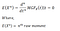Figure 10: Taking the n-th derivative of a Moment Generating Function.

a) Finding the first raw moment:

b) Finding the second raw moment:

# Proof: n-th derivative of MGF is the n-th moment

Here we will use Taylor’s series to prove it.

From that, we can say that,

Finding the expected value of e^tx:

Now let us prove that the n-th derivative of E(e^tx) is nth-moment.

a) Finding the first derivative: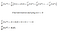Figure 16: Taking the first derivative of a Moment Generating Function.

Here we can see that it gives us the first moment.

b) Finding the second derivative: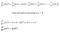Figure 17: Taking the second derivative of a Moment Generating function.

Here we can see that it gives us a second moment.

From these two derivations, we can confidently say that the nth-derivative of Moment Generating Function is the nth-moment.

# What is the role of “t” in Moment Generating Function?

From the above derivations, we can see that the variable “t” works as a helper variable. By using “t,” we can find different derivatives in Moment Generating Function.

# Why do we need MGF?

In the case of a continuous probability distribution, we have to integrate the Probability Density Function(PDF) to find the moments of a distribution. Moreover, it turns out that finding integration adds complexity to an algorithm and increases the run time of a program. As an alternative to that, we use Moment Generating Functions and their derivations to find the moments. Please note that we can get the moments without using Moment Generating Function, but it gets complicated as we move forward to calculate higher-order moments.

# Relationship between Raw and Central moments:

At this point, we know that,

Now we will find the relationship between the central moment and raw moment.

a) Write the formula in a different form:

b) Expanding the main term using binomial theorem:

c) Expanding our primary term:

d) Put that in the main formula:

e) Simplifying the terms using the definition of the raw moment: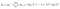Figure 24: The centered moment in terms of the raw moment.

f) Write the formula in a simple form:

Voila! We have derived the formula to find the relationship between raw moments and central moments. Now let’s find the relationship between them.

a) First Central Moment in terms of Raw Moments:

2) Second Central Moment in terms of Raw Moments:

3) Third Central Moment in terms of Raw Moments: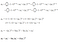Figure 28: Third central moment in terms of the raw moments.

4) Fourth Central Moment in terms of Raw Moments: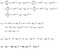Figure 29: Fourth central moment in terms of the raw moments.

In summary,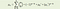Figure 30: The centered moment in terms of the raw moments.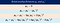Figure 31: Summary of the relationship between the centered and the raw moments.

Please note that we get the raw moments while finding the moments by Moment Generating Function(MGF). We can find out the central moments from the raw moments using the above-derived formulas. We can easily find the standardized moments using the central moments. We will use these formulas in our future tutorials on probability distributions.

# Python Implementation:

Using Python, we can find the central moments for a dataset. Let’s have a look at a few examples.

1) 1-Dimensional Data:

2) 2-Dimensional Data:

3) 2-Dimensional Data with axis=1:

4) Multi-Dimensional Data:

5) Higher-Order Moments:

1. For any valid Moment Generating Function, we can say that the 0th moment will be equal to 1.
2. Finding the derivatives using the Moment Generating Function gives us the Raw moments.
3. Once we have the MGF for a probability distribution, we can easily find the n-th moment.
4. Each probability distribution has a unique Moment Generating Function.
5. We can find moments without using Moment Generating Function, but using MGF reduces the time and space complexity.

In future articles, we will see each probability distributions in detail with their Moment Generating Function. We will use the derived formulas from this piece in those tutorials. Any suggestions or feedback is crucial to continue to improve. Please let us know in the comments if you have any.

DISCLAIMER: The views expressed in this article are those of the author(s) and do not represent the views of Carnegie Mellon University. These writings do not intend to be final products, yet rather a reflection of current thinking, along with being a catalyst for discussion and improvement.

Published via Towards AI

 scipy.stats.moment, SciPy.org, https://docs.scipy.org/doc/scipy/reference/generated/scipy.stats.moment.html

 Moments in Statistics: Definition, Examples, Statistics How To, https://www.statisticshowto.com/what-is-a-moment/

 Moment-generating Function, Wikipedia, https://en.wikipedia.org/wiki/Moment-generating_function

 Taylor Series, Wikipedia, https://en.wikipedia.org/wiki/Taylor_series

 Binomial Theorem, Wikipedia, https://en.wikipedia.org/wiki/Binomial_theorem

## Towards AI

### By Towards AI

Towards AI publishes the best of tech, science, and engineering. Subscribe with us to receive our newsletter right on your inbox. For sponsorship opportunities, please email us at pub@towardsai.net Take a look

Written by

Written by

## Towards AI Team

#### Publishing the Best of Tech, Science, and Engineering | Editorial → https://towardsai.net/p/editorial | Subscribe→ https://towardsai.net/subscribe — @Towards_AI